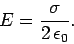Energy and Work Energy and Work Physics Help ForumApr 5th 2013, 02:00 PM #1 Junior Member   Join Date: Apr 2013 Posts: 3 Distance ? Good morning/evening <3 For my problem, A metal ball with a mass of 3x10^-6 kg and a charge of +5 mC has a kinetic energy of 6x10^8 j. it's directly at an infinite plane of charge with a charge distribution of +4c/m^2. if it's currently 1m away from the plane of charge, how close will it come to the plane before stopping? (the correct answer is: 0.266 m) ----------------------------------------------------------- (my attemp): 1- finding v2 from Ek = ½mv2 I get v= 20x10^6 m/s 2- F=ma qE=ma a= qE/m 3- find E by := 2.26x10^11 4- a=3.77x10^14 m/s^s 5- finally: vf2 = vi2 + 2ad d= vi2 /2a = 0.530 m so, 1-0.530 = 0.47m could any one tell me what's wrong?Apr 5th 2013, 03:08 PM #2 Physics Team   Join Date: Jun 2010 Location: Morristown, NJ USA Posts: 2,344 Given the data you provided, I believe your answer is correct. A way to do this that offers a slight shortcut is this: Work = change in KE, and work = Force x distance, so distance = change in KE/force. Change in KE is 6E+8 Joules Force = Eq = 1.13E+9 N So d= 6E+8 Joules/1.13E+9 N = 0.531 m. And as you say, 1 - 0.531m = 0.469mApr 5th 2013, 03:18 PM   #3
Member

Join Date: Feb 2013
Location: NM
Posts: 94
 Originally Posted by SaloomGood morning/evening <3 For my problem, A metal ball with a mass of 3x10^-6 kg and a charge of +5 mC has a kinetic energy of 6x10^8 j. it's directly at an infinite plane of charge with a charge distribution of +4c/m^2. if it's currently 1m away from the plane of charge, how close will it come to the plane before stopping? (the correct answer is: 0.266 m) ----------------------------------------------------------- (my attemp): 1- finding v2 from Ek = ½mv2 I get v= 20x10^6 m/s 2- F=ma qE=ma a= qE/m 3- find E by := 2.26x10^11.....UNITS? Is it V/m ? 4- a=3.77x10^14 m/s^s 5- finally: vf2 = vi2 + 2ad d= vi2 /2a = 0.530 m so, 1-0.530 = 0.47m could any one tell me what's wrong?The current kinetic energy (when 1 m from the plate) is 6×10^8 J. I don't think you have to find the velocity .. just use Conservation of Energy.

The change of Kinetic Energy is -6×10^8 J when the velocity has fallen to zero.
The change of electric potential is deltaV = E*d (Volts)
so multiply by Q to get the change of potential energy (V*C = J);
Q*E*d = 6×10^8 J
The only unknown is d.

I get d=0.531 m .. same as you .. final position 0.469 m from plate

Have you checked that all the numbers are as given? Why do you believe the correct answer is 0.266 m?
__________________
DrPhil (not the TV guy)
If we knew what we were doing, we wouldn't have to do it.Tags distanceThread ToolsShow Printable VersionEmail this Page Display ModesLinear ModeSwitch to Hybrid ModeSwitch to Threaded ModeSimilar Physics Forum Discussions Thread Thread Starter Forum Replies Last Post stealth Kinematics and Dynamics 5 Mar 13th 2010 10:47 AM juggalomike Advanced Mechanics 1 Jan 14th 2010 05:49 AM Book Periodic and Circular Motion 1 Dec 7th 2009 10:22 PM christina Kinematics and Dynamics 2 Nov 30th 2009 12:20 AM christina Kinematics and Dynamics 2 Oct 28th 2009 06:26 PM